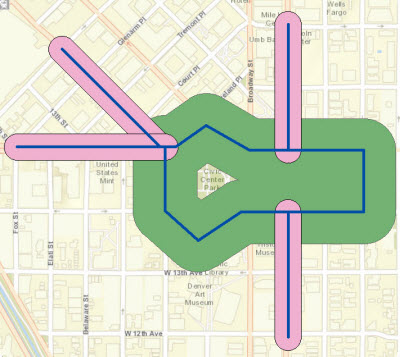The Buffer operator creates a buffer polygon around the input geometry at a specified distance. If the input geometry is a polygon and a negative distance is specified, then the buffer polygon could be inside the input geometry. The specified distance is in the units of the associated spatial reference.

Let's look at a couple of simple examples for different geometry types. In the following images, the blue geometry is the input geometry and the green geometry is a possible buffer polygon.

### Point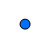Input Geometry Buffer Polygon Both

### Polyline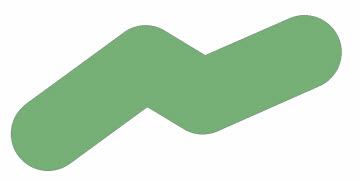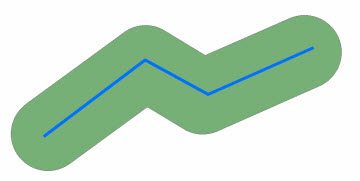Input Geometry Buffer Polygon Both

### Polygon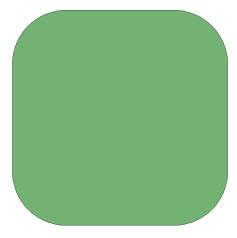Input Geometry Buffer Polygon Both

We can create buffers from much more complicated geometries too. For example, consider the input geometry shown below which represents Indonesia. This multipart polygon has 137 parts and 9,164 vertices.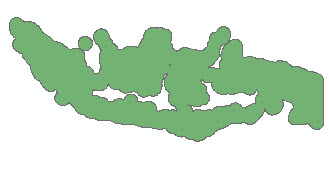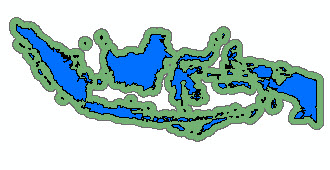Input Geometry Buffer Polygon Both

We can also buffer more than one geometry and input more than one distance parameter in a single execution by using cursors. We will get to that later.

For now, we will look at some code to generate a buffer from a single input geometry.

## Buffering a single geometry

To buffer a single geometry, we use one of the `execute` methods of the `OperatorBuffer` class.

`public abstract Geometry execute(Geometry inputGeometry, SpatialReference sr, double distance, ProgressTracker progressTracker);`

To call the `execute` method, create a local instance of the Buffer operator.

`Geometry outputGeometry = OperatorBuffer.local().execute(inputGeometry, sr, distance, null);`

### Example

The program given below creates a polygon that is then used as input to the Buffer operator. The spatial reference is GCS_WGS_1984 so the distance units are degrees.

```package geometryapp;

import com.esri.core.geometry.Geometry;
import com.esri.core.geometry.OperatorBuffer;
import com.esri.core.geometry.OperatorExportToJson;
import com.esri.core.geometry.Polygon;
import com.esri.core.geometry.SpatialReference;

/*
* This program creates a polygon, performs the Buffer operation and
* prints out the result in JSON format.
*/

public class BufferApp_1 {

public static void main(String[] args) {

Polygon poly1 = createPolygon1();

// Create a spatial reference object for GCS_WGS_1984.
SpatialReference spatialRef = SpatialReference.create(4326);

// Buffer the geometry by a distance = 0.5.
Geometry outputGeom = OperatorBuffer.local().execute(poly1, spatialRef, 0.5, null);
printResult(spatialRef, outputGeom, 0.5);

// Buffer the geometry by a distance = 0.1.
outputGeom = OperatorBuffer.local().execute(poly1, spatialRef, 0.1, null);
printResult(spatialRef, outputGeom, 0.1);

// Buffer the geometry by a distance = -0.09.
// Buffer polygon should be inside the input geometry.
outputGeom = OperatorBuffer.local().execute(poly1, spatialRef, -0.09, null);
printResult(spatialRef, outputGeom, -0.09);
}

public static Polygon createPolygon1() {

Polygon poly = new Polygon();

// Outer ring
poly.startPath(0, 0);
poly.lineTo(0, 1);
poly.lineTo(1, 1);
poly.lineTo(1, 0);

// Hole
poly.startPath(0.25, 0.25);
poly.lineTo(0.75, 0.25);
poly.lineTo(0.75, 0.75);
poly.lineTo(0.25, 0.75);

return poly;
}

public static void printResult(SpatialReference spatialRef, Geometry geometry, double distance) {

// Export the geometry to JSON format to print it out.
String jsonString = OperatorExportToJson.local().execute(spatialRef, geometry);
System.out.println("Buffer distance = " + Double.toString(distance) + ":");
System.out.println(jsonString);
System.out.println();
}
}
```

The input geometry and the buffer polygons generated by `BufferApp_1` are shown below.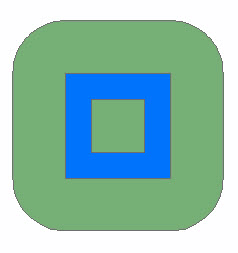Input Geometry Buffer Polygon (distance = 0.5) Both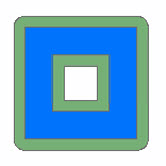Input Geometry Buffer Polygon (distance = 0.1) Both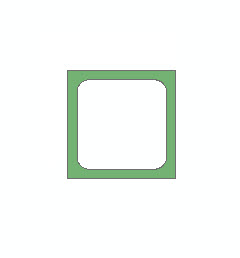Input Geometry Buffer Polygon (distance = -0.09) Both

## Buffering multiple geometries

Suppose we have many geometries for which we want to generate buffer polygons. Indeed, maybe we want the union of the resultant buffer polygons. Maybe we have a need to chain operations, that is, use the output from the Buffer operation as input to another operation. If the input geometries are the same type, it is not necessary to process them one at a time. In these and similar cases, we consider using a geometry cursor.

To buffer multiple geometries at once, we use another of the `execute` methods from the `OperatorBuffer` class.

`public abstract GeometryCursor execute(GeometryCursor inputGeometries, SpatialReference sr, double[] distances, boolean bUnion, ProgressTracker progressTracker);`

To call the `execute` method, create a local instance of the Buffer operator.

`GeometryCursor outputGeometries = OperatorBuffer.local().execute(inputGeometries, sr, distances, bUnion, null);`

Notice that we now have an array of distances. The first input geometry will be buffered using the first distance in the array, the second input geometry will be buffered using the second distance in the array and so on. If the size of the distances array is less than the number of input geometries, the last distance value in the array will be used to buffer the remaining input geometries.

The `bUnion` parameter is a boolean signifying whether the buffer polygons should be unioned or not. If `bUnion` is set to `true`, then the output cursor contains only one polygon, namely, the union of all the buffer polygons. If `bUnion` is set to `false`, then the output cursor contains each of the buffer polygons as an individual geometry.

### Example

Consider the polylines shown below representing a portion of downtown Denver streets.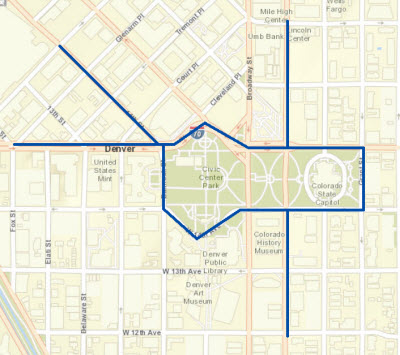We would like a buffer of 120 meters around the closed polyline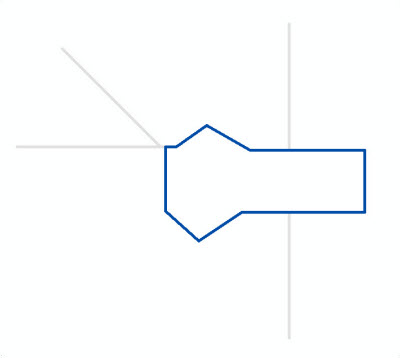and a buffer of 50 meters around the remaining polylines.We can accomplish our task with the program given below. It creates the five polylines and a geometry cursor that is used as input to the Buffer operator. The first polyline is buffered by a distance of 120 meters and the remaining polylines by 50 meters. The spatial reference is NAD_1983_2011_UTM_Zone_13N so the distance units are meters.

```package geometryapp;

import com.esri.core.geometry.Geometry;
import com.esri.core.geometry.GeometryCursor;
import com.esri.core.geometry.OperatorBuffer;
import com.esri.core.geometry.OperatorExportToJson;
import com.esri.core.geometry.Polyline;
import com.esri.core.geometry.SimpleGeometryCursor;
import com.esri.core.geometry.SpatialReference;
import java.util.ArrayList;

/*
* This program creates a cursor with five polylines to be used as input to the
* Buffer operator. The first polyline is buffered by 120 meters and the remaining
* polylines are buffered by 50 meters. The results are printed out in JSON format.
*/

public class BufferApp_2 {

public static void main(String[] args) {

// Create the input polylines to buffer.
ArrayList<Geometry> geomList = createAllPolylines();

// Create the geometry cursor from the list of polylines.
SimpleGeometryCursor inputGeoms = new SimpleGeometryCursor(geomList);

// Create a spatial reference object for NAD_1983_2011_UTM_Zone_13N.
SpatialReference spatialRef = SpatialReference.create(102382);

// Buffer the first polyline by a distance = 120 and the rest by a distance = 50.
double distances[] = {120, 50};

// Buffer the geometries. Do not union the results.
GeometryCursor outputGeoms = OperatorBuffer.local().execute(inputGeoms, spatialRef, distances, false, null);

// Get the geometries from the cursor and print them in JSON format.
Geometry geom = null;
while((geom = outputGeoms.next()) != null) {
printResult(spatialRef, geom);
}
}

public static ArrayList<Geometry> createAllPolylines() {

// Create a list of input geometries.
ArrayList<Geometry> geomList = new ArrayList<Geometry>(5);

// Create a list of coordinates to use for creating the polylines.
double coords[][] = {{-11686713,4828005},{-11687175,4828005},{-11687337,4827898},
{-11687461,4828009},{-11687461,4828250},{-11687421,4828250},{-11687305,4828331},
{-11687143,4828237},{-11686716,4828237},{-11686713,4828237},{-11686713,4828005}};

double coords2[][] = {{-11686998,4828712},{-11686998,4828240}};

coords2 = -11686998;
coords2 = 4828001;
coords2 = -11686998;
coords2 = 4827533;

coords2 = -11687848;
coords2 = 4828618;
coords2 = -11687480;
coords2 = 4828251;

coords2 = -11688017;
coords2 = 4828250;
coords2 = -11687461;
coords2 = 4828250;

return geomList;
}

public static Polyline createPolyline(double[][] pts) {

Polyline line = new Polyline();

line.startPath(pts, pts);

for (int i = 1; i < pts.length; i++)
line.lineTo(pts[i], pts[i]);

return line;
}

public static void printResult(SpatialReference spatialRef, Geometry geometry) {

// Export the geometry to JSON format to print it out.
String jsonString = OperatorExportToJson.local().execute(spatialRef, geometry);
System.out.println(jsonString);
System.out.println();
}
}
```

The input geometries and the buffer polygons generated by `BufferApp_2` are shown below.